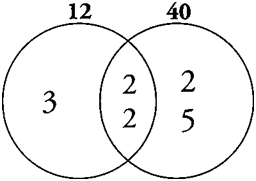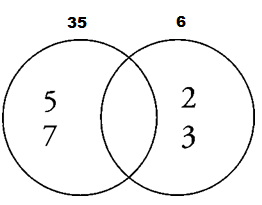Option Training Institute FZ LLC G 37 & G 34, Block 13, Al Sufouh Road Knowledge, Village Dubai PO Box : 501703

# Few Factors, More MultiplesContinuing our discussion of prime numbers, in this post we shall discuss two very important concepts of GCF or LCM. Frequently on the GMAT, you may have to find the Greatest Common Factor (GCF) or Least Common Multiple (LCM) of a set of two or more numbers.

Greatest Common Factor (GCF): the largest factor (or divisor) of two or more integers. Also known as GCD (Greatest Common Divisor)

Least Common Multiple (LCM): the smallest multiple of two or more integers.

Please not that the LCM, because it is a multiple, will always be larger than or equal to the starting integers. GCF will be smaller than or equal to the starting integers because we are talking about a factor (The first word “least” and “greatest” in both cases is slightly misleading. What really matters is that LCM is a multiple while GCF is a factor).

Finding GCF and LCM Using Venn Diagrams

To find the GCF and LCM of two numbers using Venn Diagrams, perform the following steps

1) Find the prime factorization

2) Put the results in a Venn diagram

3) The shared numbers go in the shared space (middle) of the Venn diagram

4) Place the remaining (non-common) factors in the non-shared areas

5) Multiply the shared numbers together = GCF

6) Multiply all the prime numbers in the diagram = LCM

E.g. to compute the GCF and LCM of 12 and 40 using the Venn diagram approach.First find the prime factorizations. 12=22 x 3. 40=23 x 5. The only common factors of 12 and 40 are the two 2’s. Therefore, place two 2’s in the shared area of the Venn diagram and remove them from both prime factorizations. Then, place the remaining factors in the unshared areas

The GCF of 12 and 40 is therefore 2×2 = 4, the prod­uct of the primes in the shared area. (An easy way to remember this is that the “common factors” are in the common area.)The LCM is2x2x2x3x5 = 120, the product of all the primes in the diagram.

Note that if two numbers have no primes in common, then their GCF is 1 and their LCM is simply their product. For example, 35 (= 5 x 7) and 6 (= 2 x 3) have no common prime numbers. Therefore, their GCF is 1 and their LCM is 35 x 6 = 210.

Be careful: even though you have no primes in the common area, the GCF is not 0 but 1.Now let’s have a look at how GCF and LCM are useful in solving GMAT questions.

Question – There are 16 girls and 40 boys in a group. What is the minimum number of equal sized rows in which they can be made to stand if a row must contain either girls or boys but not both?

Solution – Before we get to the number of rows, it is important to get the maximum size of each row. The maximum size of each row is the GCF of 16 and 40 (8). If you happen to get confused between using GCF and LCM, just remember that GCF is either equal to smaller number or even smaller. Once we have size of each row (the GCF), number of rows of girls = 2 (16/8) and number of rows of boys = 5 (40/8). Hence, total number of rows = 2+5 = 7.

Question – The number of cartons in a coastal store can be divided evenly into 25 equal shipments by ship or 15 equal shipments by boat. What is the smallest number of cartons that could be in the store?

Solution – The question says that the number of cartons is evenly divisible by both 25 and 15; in other words, it’s a common multiple of 25 and 15. Again, in case you are confused, just remember that LCM gives a value which is equal to or bigger than the greater of the two numbers. It is clear that number of cartons must be greater than 25 and 15, hence answer is their LCM = 75.

Here’s a data sufficiency question for you based on the concept of LCM and GCF.

Question – The integers m and p are such that 2 is less than m and m is less than p. Also, m is not a factor of p. If r is the remainder when p is divided by m, is r > 1.

1. The greatest common factor of m and p is 2.

2. The least common multiple of m and p is 30.

At Option Training Institute, our constant endeavor is to help aspirants do well in Quant even if they found Math cryptic in high school.